# Telangana SSC Board (TS SSC) Question Paper 2 for Class 10th Maths 2018 In PDF

## Telangana 10th Annual Exam Question Papers 2018 Maths Paper 2 with Solutions – Free Download

Telangana SSC Class 10 Maths 2018 Question Paper 2 with solutions are given at BYJU’S in a downloadable pdf format, which will help the students to access at any time easily. Students can also go through with the Telangana Class 10 Maths previous year question papers, this will help in analysing the exam pattern for the upcoming exams conducted by the board

They can download the Telangana SSC question paper 2018 Maths Paper 2 to practice as part of their preparation. BYJU’S provides solutions for all these papers, so that students can verify them whenever required. Telangana SSC question paper 2018 Maths Paper 2 solutions will help the students to excel their problem-solving skills and analysing skills.

### Telangana Board SSC Class 10 Maths 2018 Question Paper 2 with Solutions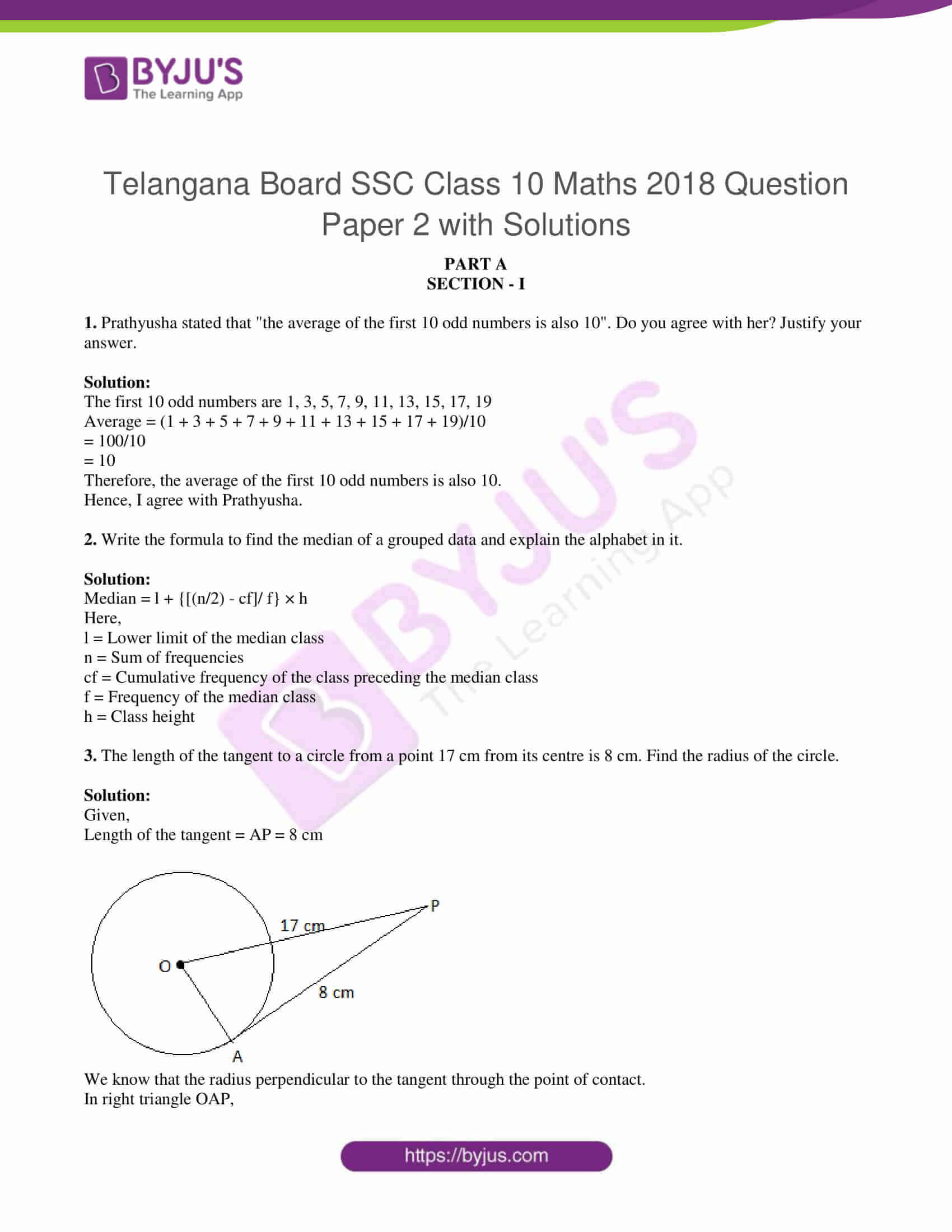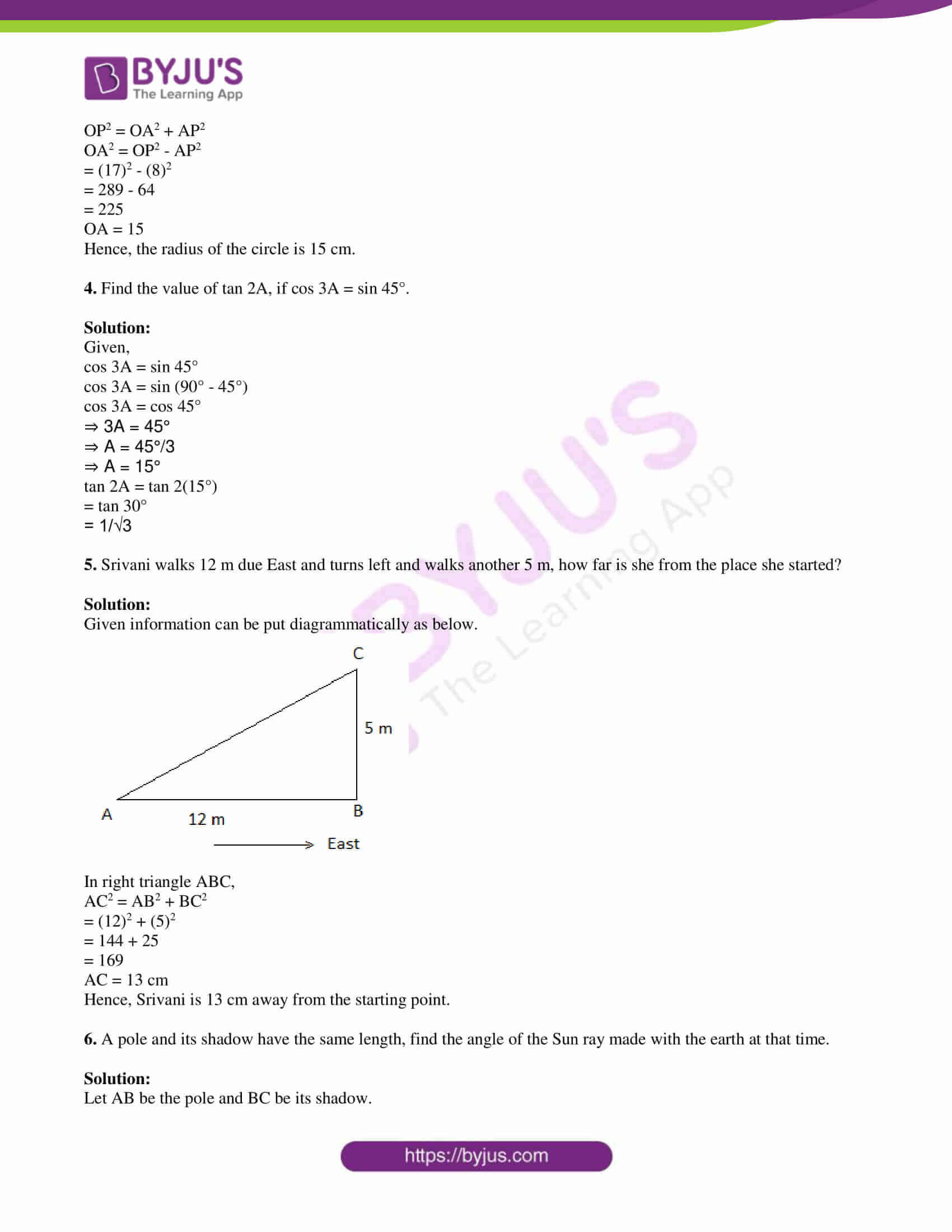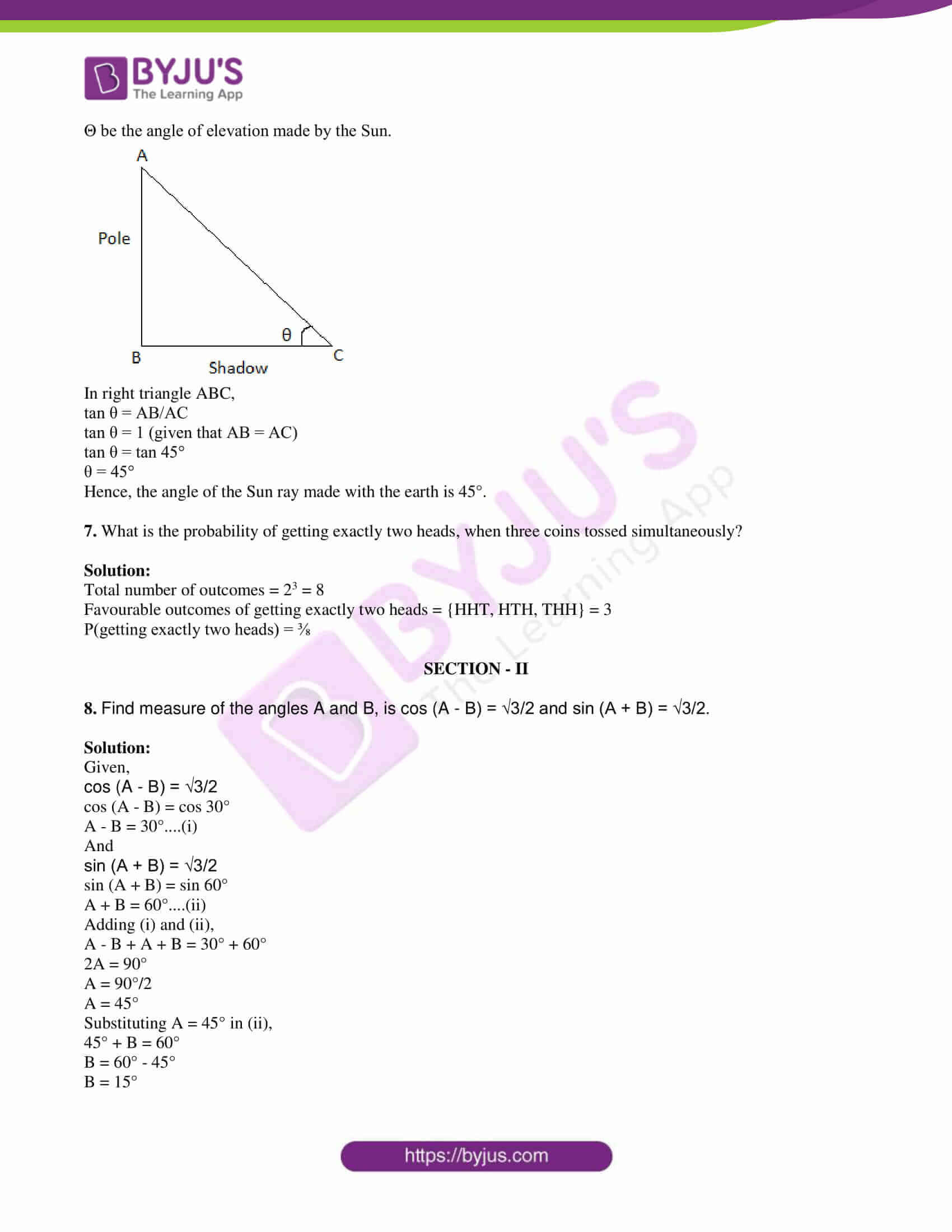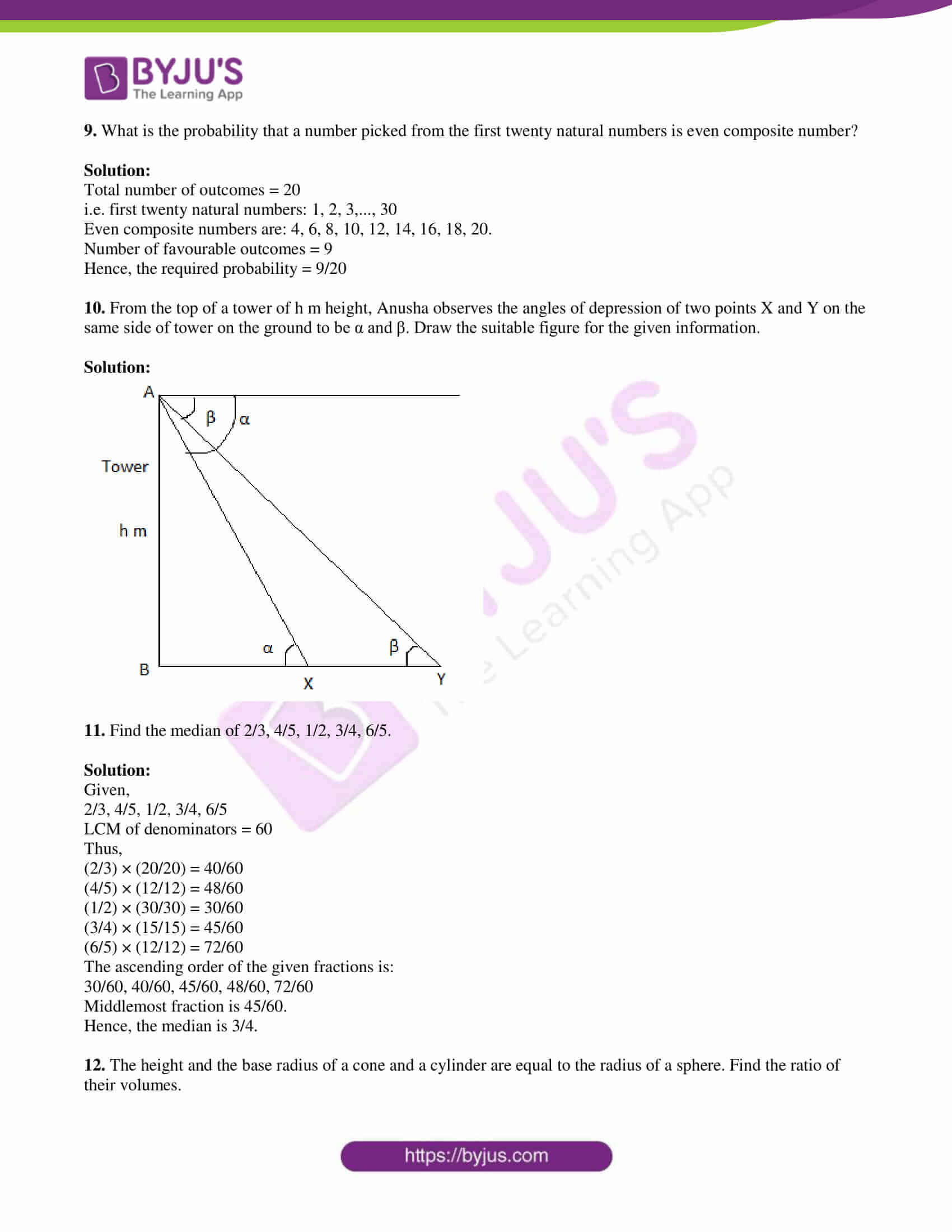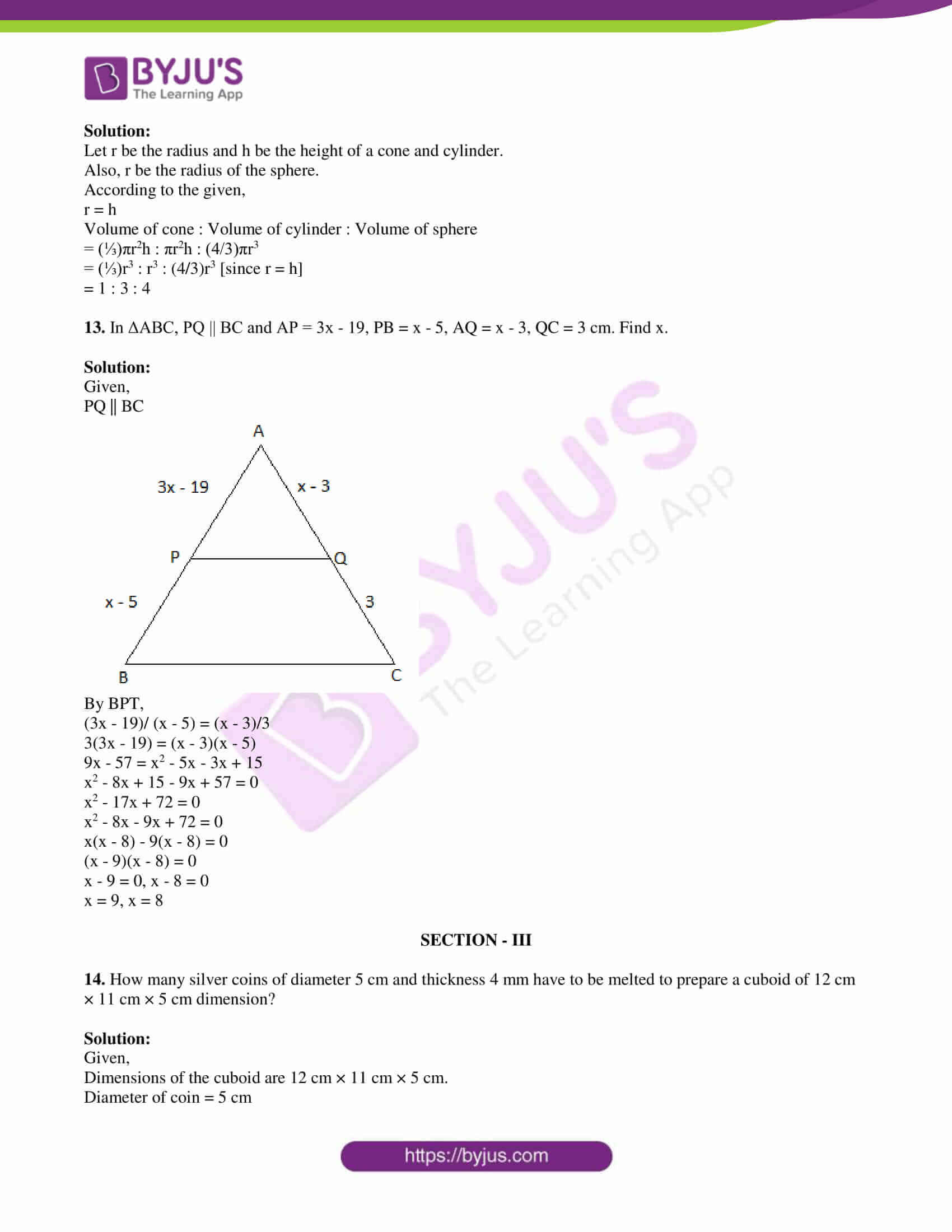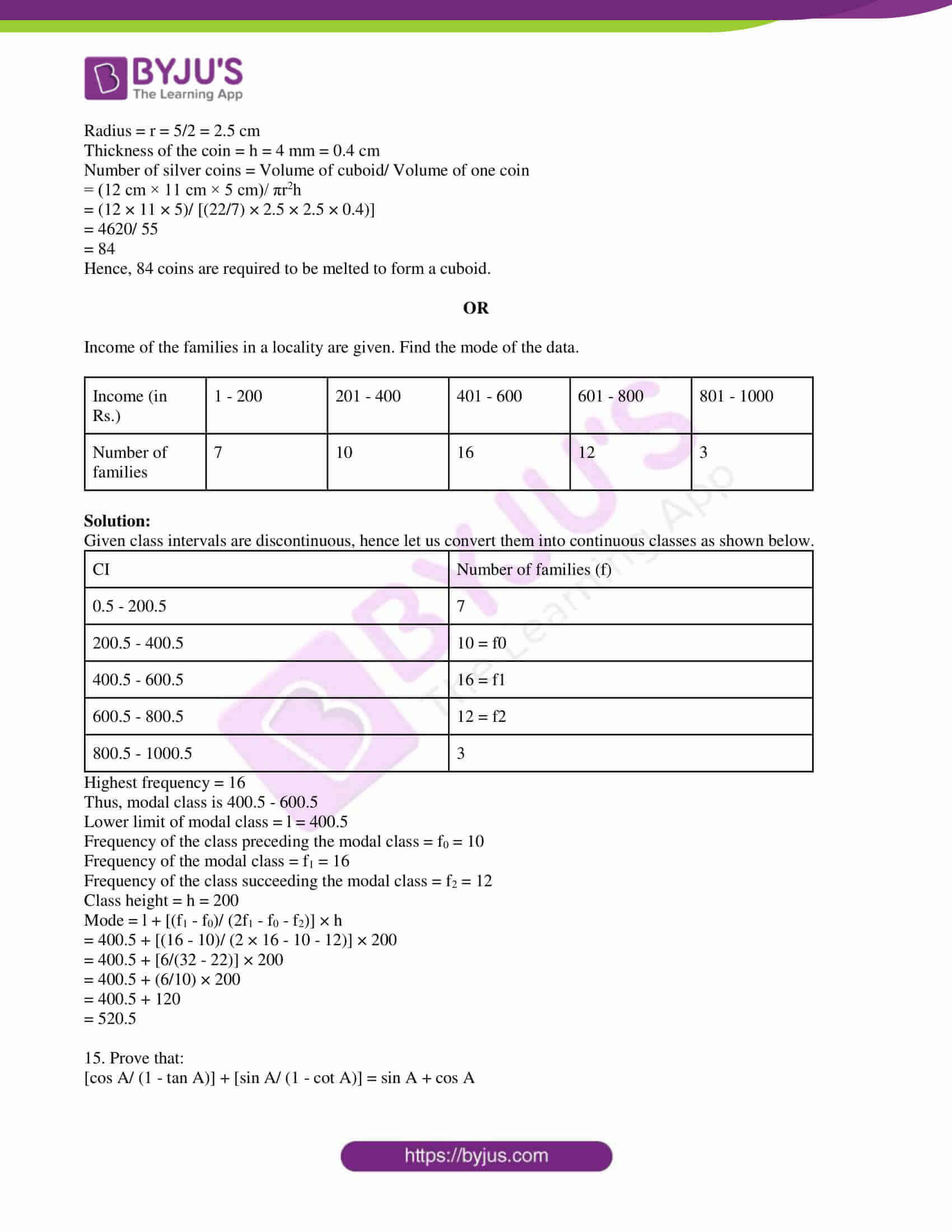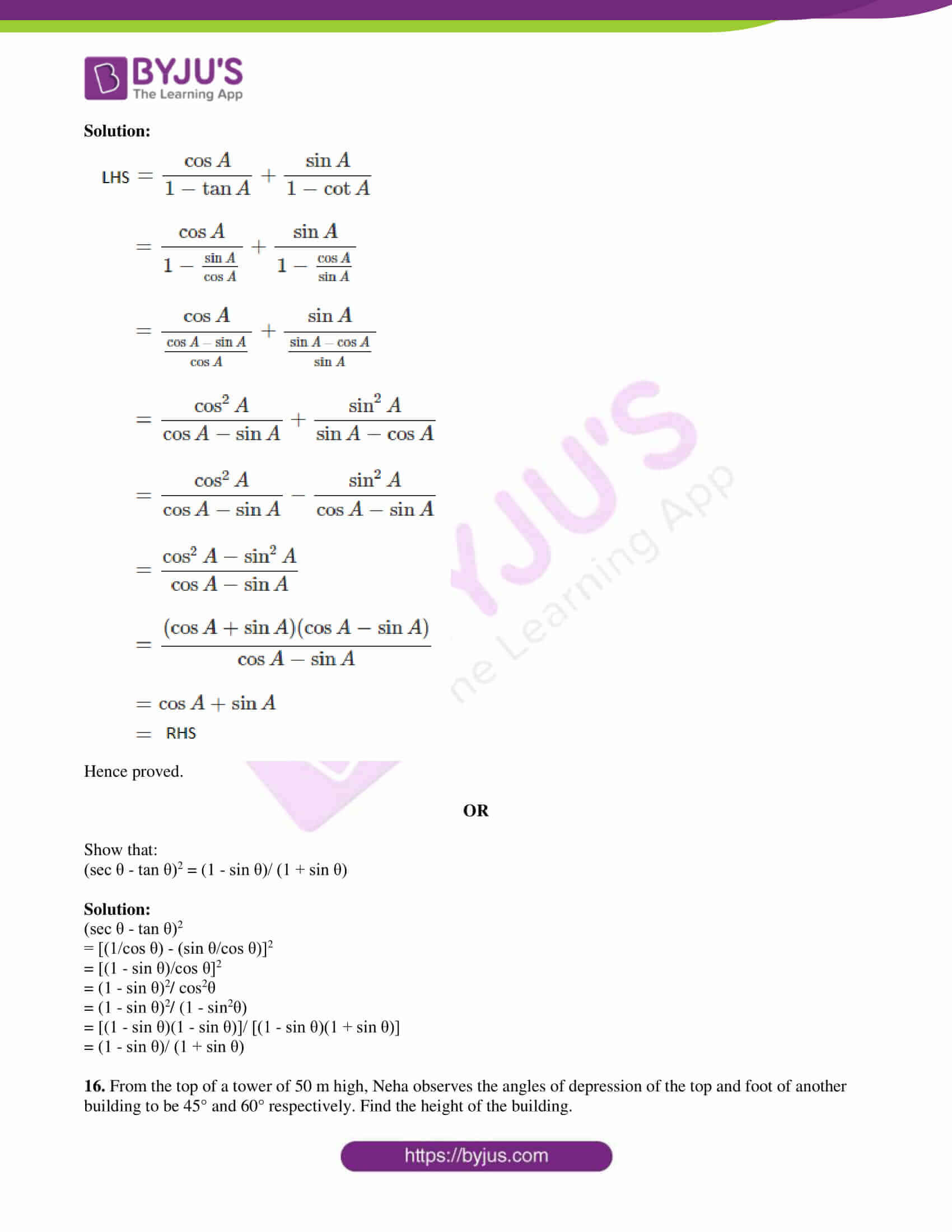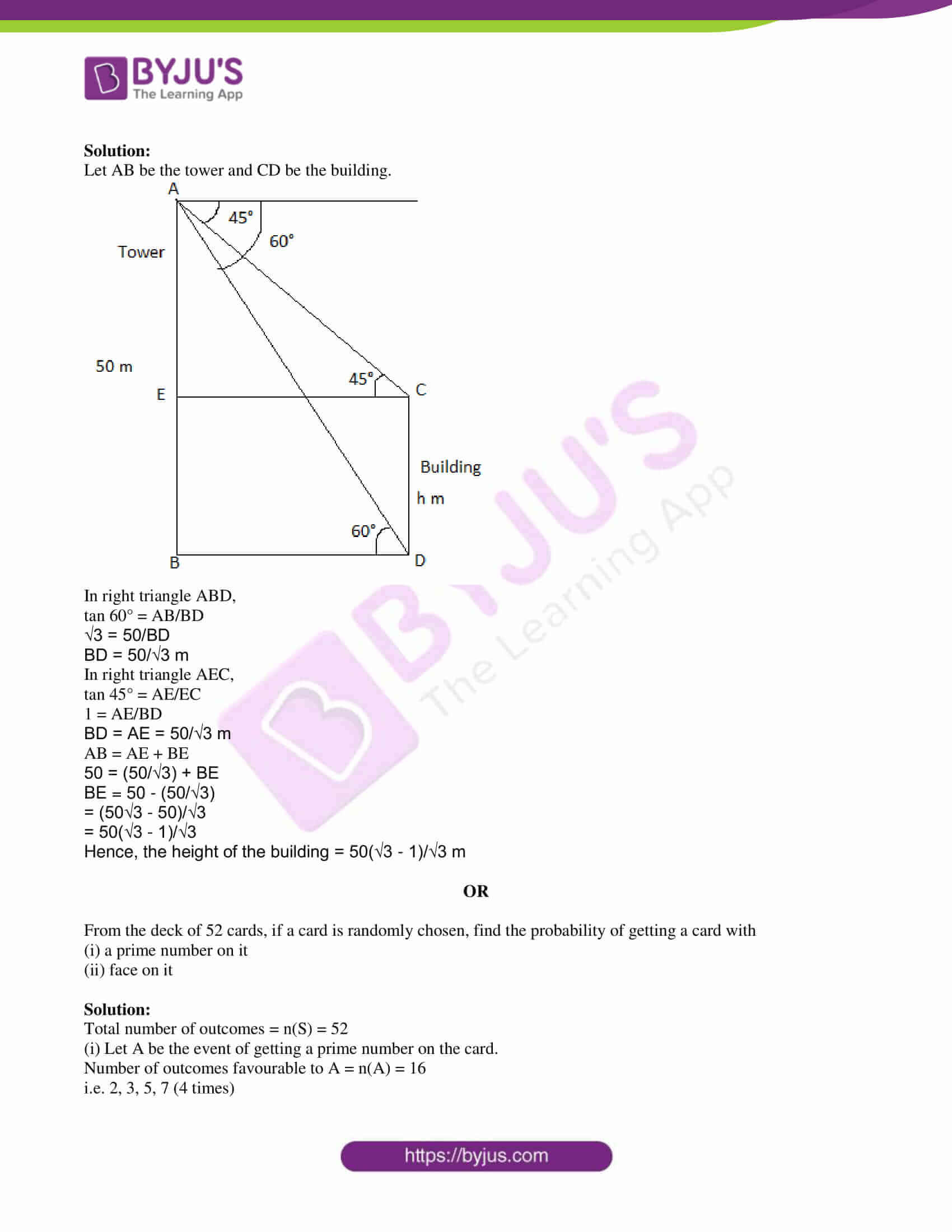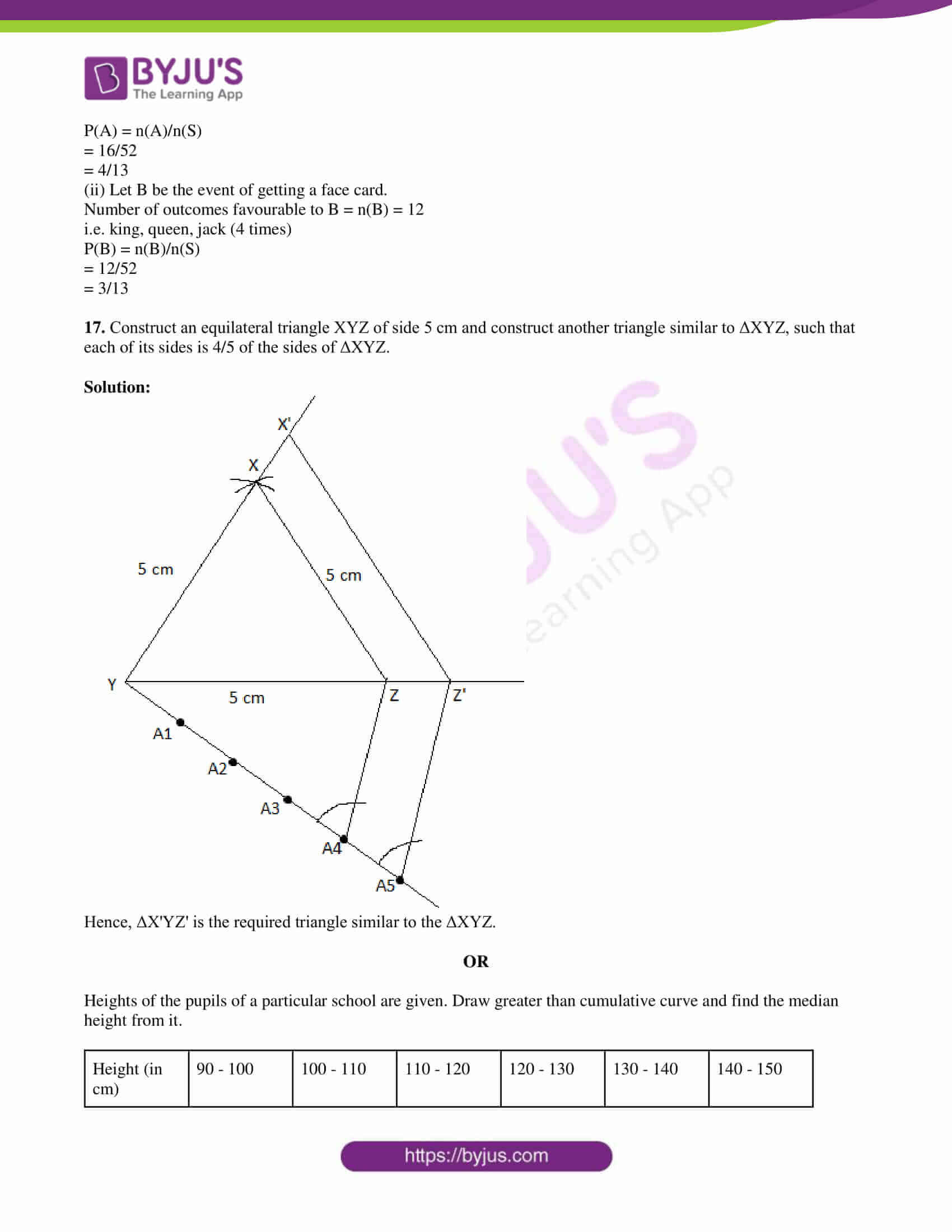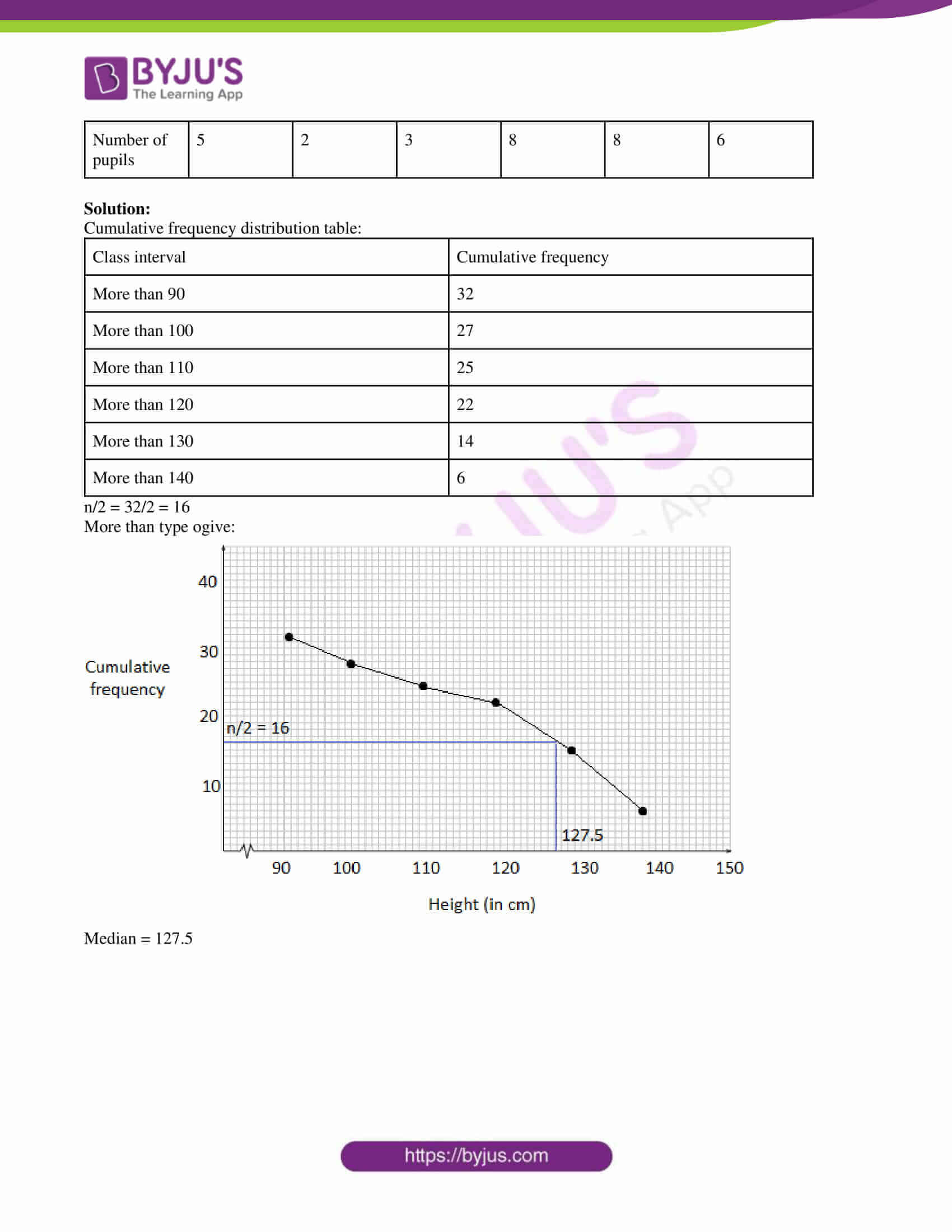PART A

SECTION – I

1. Prathyusha stated that “the average of the first 10 odd numbers is also 10”. Do you agree with her? Justify your answer.

Solution:

The first 10 odd numbers are 1, 3, 5, 7, 9, 11, 13, 15, 17, 19

Average = (1 + 3 + 5 + 7 + 9 + 11 + 13 + 15 + 17 + 19)/10

= 100/10

= 10

Therefore, the average of the first 10 odd numbers is also 10.

Hence, I agree with Prathyusha.

2. Write the formula to find the median of a grouped data and explain the alphabet in it.

Solution:

Median = l + {[(n/2) – cf]/ f} × h

Here,

l = Lower limit of the median class

n = Sum of frequencies

cf = Cumulative frequency of the class preceding the median class

f = Frequency of the median class

h = Class height

3. The length of the tangent to a circle from a point 17 cm from its centre is 8 cm. Find the radius of the circle.

Solution:

Given,

Length of the tangent = AP = 8 cm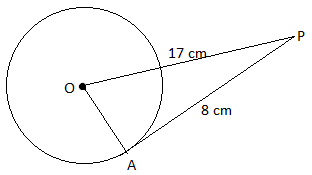We know that the radius perpendicular to the tangent through the point of contact.

In right triangle OAP,

OP2 = OA2 + AP2

OA2 = OP2 – AP2

= (17)2 – (8)2

= 289 – 64

= 225

OA = 15

Hence, the radius of the circle is 15 cm.

4. Find the value of tan 2A, if cos 3A = sin 45°.

Solution:

Given,

cos 3A = sin 45°

cos 3A = sin (90° – 45°)

cos 3A = cos 45°

⇒ 3A = 45°

⇒ A = 45°/3

⇒ A = 15°

tan 2A = tan 2(15°)

= tan 30°

= 1/√3

5. Srivani walks 12 m due East and turns left and walks another 5 m, how far is she from the place she started?

Solution:

Given information can be put diagrammatically as below.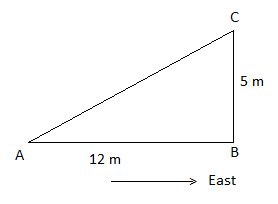In right triangle ABC,

AC2 = AB2 + BC2

= (12)2 + (5)2

= 144 + 25

= 169

AC = 13 cm

Hence, Srivani is 13 cm away from the starting point.

6. A pole and its shadow have the same length, find the angle of the Sun ray made with the earth at that time.

Solution:

Let AB be the pole and BC be its shadow.

Θ be the angle of elevation made by the Sun.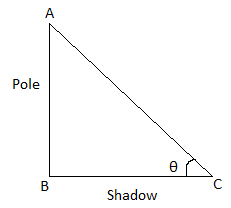In right triangle ABC,

tan θ = AB/AC

tan θ = 1 (given that AB = AC)

tan θ = tan 45°

θ = 45°

Hence, the angle of the Sun ray made with the earth is 45°.

7. What is the probability of getting exactly two heads, when three coins tossed simultaneously?

Solution:

Total number of outcomes = 23 = 8

Favourable outcomes of getting exactly two heads = {HHT, HTH, THH} = 3

P(getting exactly two heads) = ⅜

SECTION – II

8. Find measure of the angles A and B, is cos (A – B) = √3/2 and sin (A + B) = √3/2.

Solution:

Given,

cos (A – B) = √3/2

cos (A – B) = cos 30°

A – B = 30°….(i)

And

sin (A + B) = √3/2

sin (A + B) = sin 60°

A + B = 60°….(ii)

A – B + A + B = 30° + 60°

2A = 90°

A = 90°/2

A = 45°

Substituting A = 45° in (ii),

45° + B = 60°

B = 60° – 45°

B = 15°

9. What is the probability that a number picked from the first twenty natural numbers is even composite number?

Solution:

Total number of outcomes = 20

i.e. first twenty natural numbers: 1, 2, 3,…, 30

Even composite numbers are: 4, 6, 8, 10, 12, 14, 16, 18, 20.

Number of favourable outcomes = 9

Hence, the required probability = 9/20

10. From the top of a tower of h m height, Anusha observes the angles of depression of two points X and Y on the same side of tower on the ground to be α and β. Draw the suitable figure for the given information.

Solution: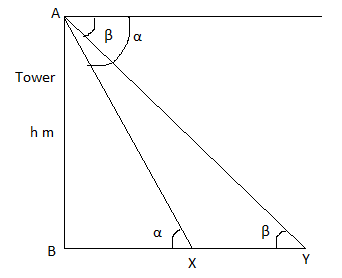11. Find the median of 2/3, 4/5, 1/2, 3/4, 6/5.

Solution:

Given,

2/3, 4/5, 1/2, 3/4, 6/5

LCM of denominators = 60

Thus,

(2/3) × (20/20) = 40/60

(4/5) × (12/12) = 48/60

(1/2) × (30/30) = 30/60

(3/4) × (15/15) = 45/60

(6/5) × (12/12) = 72/60

The ascending order of the given fractions is:

30/60, 40/60, 45/60, 48/60, 72/60

Middlemost fraction is 45/60.

Hence, the median is 3/4.

12. The height and the base radius of a cone and a cylinder are equal to the radius of a sphere. Find the ratio of their volumes.

Solution:

Let r be the radius and h be the height of a cone and cylinder.

Also, r be the radius of the sphere.

According to the given,

r = h

Volume of cone : Volume of cylinder : Volume of sphere

= (⅓)πr2h : πr2h : (4/3)πr3

= (⅓)r3 : r3 : (4/3)r3 [since r = h]

= 1 : 3 : 4

13. In ΔABC, PQ || BC and AP = 3x – 19, PB = x – 5, AQ = x – 3, QC = 3 cm. Find x.

Solution:

Given,

PQ || BCBy BPT,

(3x – 19)/ (x – 5) = (x – 3)/3

3(3x – 19) = (x – 3)(x – 5)

9x – 57 = x2 – 5x – 3x + 15

x2 – 8x + 15 – 9x + 57 = 0

x2 – 17x + 72 = 0

x2 – 8x – 9x + 72 = 0

x(x – 8) – 9(x – 8) = 0

(x – 9)(x – 8) = 0

x – 9 = 0, x – 8 = 0

x = 9, x = 8

SECTION – III

14. How many silver coins of diameter 5 cm and thickness 4 mm have to be melted to prepare a cuboid of 12 cm × 11 cm × 5 cm dimension?

Solution:

Given,

Dimensions of the cuboid are 12 cm × 11 cm × 5 cm.

Diameter of coin = 5 cm

Radius = r = 5/2 = 2.5 cm

Thickness of the coin = h = 4 mm = 0.4 cm

Number of silver coins = Volume of cuboid/ Volume of one coin

= (12 cm × 11 cm × 5 cm)/ πr2h

= (12 × 11 × 5)/ [(22/7) × 2.5 × 2.5 × 0.4)]

= 4620/ 55

= 84

Hence, 84 coins are required to be melted to form a cuboid.

OR

Income of the families in a locality are given. Find the mode of the data.

 Income (in Rs.) 1 – 200 201 – 400 401 – 600 601 – 800 801 – 1000 Number of families 7 10 16 12 3

Solution:

Given class intervals are discontinuous, hence let us convert them into continuous classes as shown below.

 CI Number of families (f) 0.5 – 200.5 7 200.5 – 400.5 10 = f0 400.5 – 600.5 16 = f1 600.5 – 800.5 12 = f2 800.5 – 1000.5 3

Highest frequency = 16

Thus, modal class is 400.5 – 600.5

Lower limit of modal class = l = 400.5

Frequency of the class preceding the modal class = f0 = 10

Frequency of the modal class = f1 = 16

Frequency of the class succeeding the modal class = f2 = 12

Class height = h = 200

Mode = l + [(f1 – f0)/ (2f1 – f0 – f2)] × h

= 400.5 + [(16 – 10)/ (2 × 16 – 10 – 12)] × 200

= 400.5 + [6/(32 – 22)] × 200

= 400.5 + (6/10) × 200

= 400.5 + 120

= 520.5

15. Prove that:

[cos A/ (1 – tan A)] + [sin A/ (1 – cot A)] = sin A + cos A

Solution: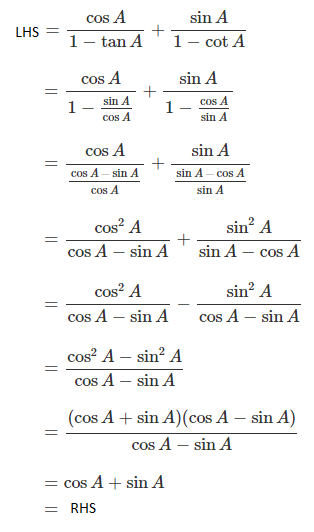Hence proved.

OR

Show that:

(sec θ – tan θ)2 = (1 – sin θ)/ (1 + sin θ)

Solution:

(sec θ – tan θ)2

= [(1/cos θ) – (sin θ/cos θ)]2

= [(1 – sin θ)/cos θ]2

= (1 – sin θ)2/ cos2θ

= (1 – sin θ)2/ (1 – sin2θ)

= [(1 – sin θ)(1 – sin θ)]/ [(1 – sin θ)(1 + sin θ)]

= (1 – sin θ)/ (1 + sin θ)

16. From the top of a tower of 50 m high, Neha observes the angles of depression of the top and foot of another building to be 45° and 60° respectively. Find the height of the building.

Solution:

Let AB be the tower and CD be the building.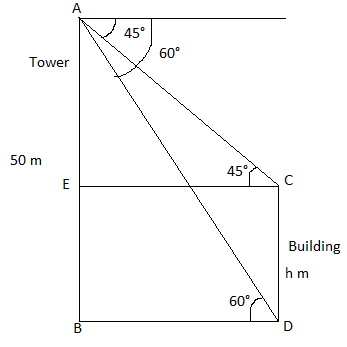In right triangle ABD,

tan 60° = AB/BD

√3 = 50/BD

BD = 50/√3 m

In right triangle AEC,

tan 45° = AE/EC

1 = AE/BD

BD = AE = 50/√3 m

AB = AE + BE

50 = (50/√3) + BE

BE = 50 – (50/√3)

= (50√3 – 50)/√3

= 50(√3 – 1)/√3

Hence, the height of the building = 50(√3 – 1)/√3 m

OR

From the deck of 52 cards, if a card is randomly chosen, find the probability of getting a card with

(i) a prime number on it

(ii) face on it

Solution:

Total number of outcomes = n(S) = 52

(i) Let A be the event of getting a prime number on the card.

Number of outcomes favourable to A = n(A) = 16

i.e. 2, 3, 5, 7 (4 times)

P(A) = n(A)/n(S)

= 16/52

= 4/13

(ii) Let B be the event of getting a face card.

Number of outcomes favourable to B = n(B) = 12

i.e. king, queen, jack (4 times)

P(B) = n(B)/n(S)

= 12/52

= 3/13

17. Construct an equilateral triangle XYZ of side 5 cm and construct another triangle similar to ΔXYZ, such that each of its sides is 4/5 of the sides of ΔXYZ.

Solution: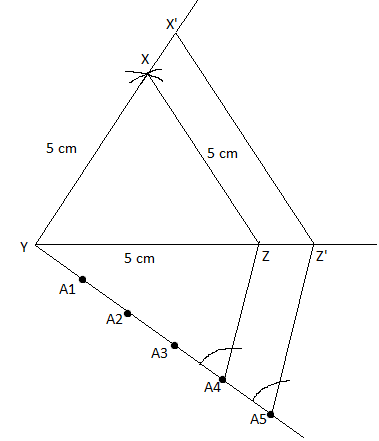Hence, ΔX’YZ’ is the required triangle similar to the ΔXYZ.

OR

Heights of the pupils of a particular school are given. Draw greater than cumulative curve and find the median height from it.

 Height (in cm) 90 – 100 100 – 110 110 – 120 120 – 130 130 – 140 140 – 150 Number of pupils 5 2 3 8 8 6

Solution:

Cumulative frequency distribution table:

 Class interval Cumulative frequency More than 90 32 More than 100 27 More than 110 25 More than 120 22 More than 130 14 More than 140 6

n/2 = 32/2 = 16

More than type ogive: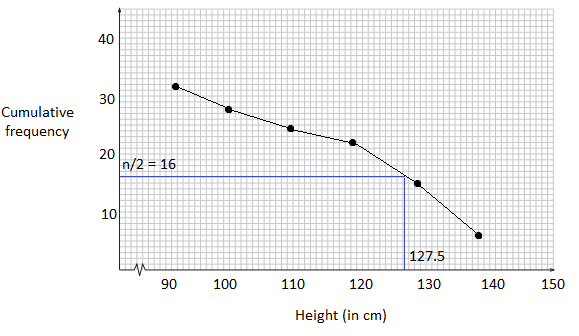Median = 127.5